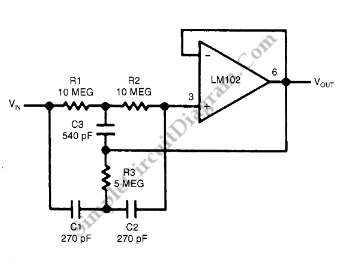# High-Q Notch Filter with Active Twin T Network

This is a circuit of High-Q Notch Filter. This circuit consist of LM102 and a twin “T” network. Twin T network sum two signal from different path with different phase shift. At certain frequency, the phase shift will be 180 degrees.  The sum will produce zero output since one signal is the inverse of other signal, in other words, the network remove signal component at certain frequency. The junction of C3 and R3 is bootstrapped to follower’s output. The frequency and depth of notch will not change because of the very low impedance of follower’s output. However, the feedback signal will increase the Q factor. Here is the schematic diagram of the circuit:The F0 is determined by following equation:

f0=1/(2*π*R1*C1)=60Hz